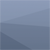A Polar Graph

I wanted to illustrate the relationship between the graph of the function r=f(θ) and the polar curve r=f(θ). In this case, the polar curve is r=0.5+1.5cos(3θ).

Source code (which is not very clean): sketch_070504a

Built with Processing